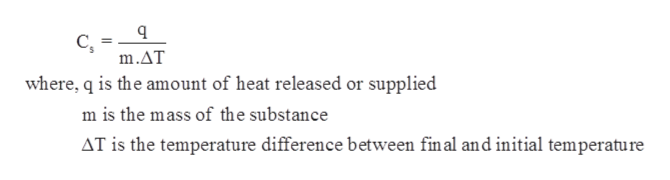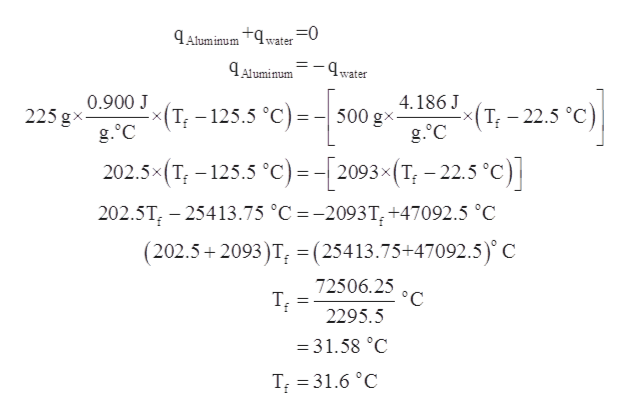# A 225 g of sample of aluminum was heated to 125.5 oC, then placed into 500.0 g of water at 22.5 oC ( specific heat of aluminum is 0.900 J/g oC). Calculate the final temperature of the mixture. (Assume no heat is lost to the surroundings). Express answer in 3 significant figures.

Question
17 views

A 225 g of sample of aluminum was heated to 125.5 oC, then placed into 500.0 g of water at 22.5 oC ( specific heat of aluminum is 0.900 J/g oC). Calculate the final temperature of the mixture. (Assume no heat is lost to the surroundings). Express answer in 3 significant figures.

check_circle

Step 1

Given information:

Mass of sample of aluminum = 225 g

Mass of water = 500.0 g

Initial temperature of a sample of aluminum = 125.5 ͦC

Initial temperature of water = 22.5 ͦC

Specific heat of aluminum = 0.900 J/g. ͦC

Step 2

The amount of heat required to raise the temperature of 1 gram of a substance by 1 ͦC is known as specific heat capacity. The expression for calculating heat capacity is represented as follows:help_outlineImage TranscriptioncloseC m.AT where, q is the amount of heat released or supplied m is the mass of the substance AT is the temperature difference between final and initial temperature fullscreen
Step 3

Assuming no heat is lost to the surroundings and taking value of specific heat of water (4.18...help_outlineImage TranscriptioncloseAluminum 9water0 4Aluminum water 4.186 J (T 125.5 °C)= -500 gx- g.°C (1-25 c) 0.900 J 225 gx g.°C 202.5x (T, -125.5 °C)- -2093x (T, - 22.5 °c) 202.5T, 25413.75 °C =-2093T,+47092.5 °C (202.5 2093)T, = (25413.75+47092.5) C 72506.25 °C 2295.5 31.58 °C T 31.6 °C fullscreen

### Want to see the full answer?

See Solution

#### Want to see this answer and more?

Solutions are written by subject experts who are available 24/7. Questions are typically answered within 1 hour.*

See Solution
*Response times may vary by subject and question.
Tagged in

### Physical Chemistry